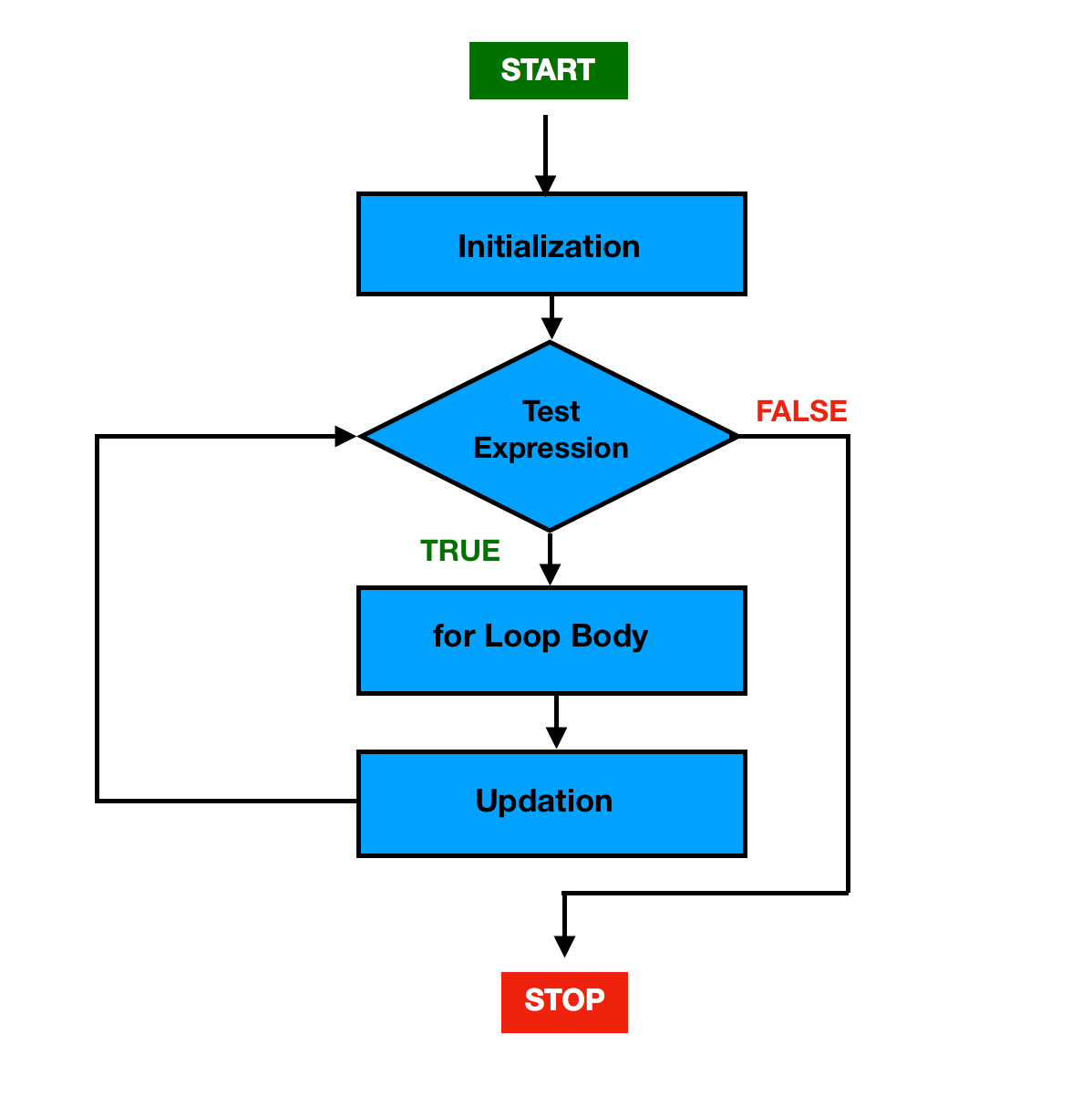# Java for Loop

In this article, you will learn about how to create for loop in Java programming with the help of examples.

Loops in Java are used when we want to execute a block of statements repeatedly until a specific condition is met(condition is false).

### Java for Loop

A for loop is used to repeat a specific block of code a known number of times.

It provides a concise way of writing the loop structure. Unlike a while loop, a for statement contains the initialization, test-expression and increment/decrement in a single line.

The for loop is mainly used when you know exactly how many times the loop is about to be executed i.e. with what values will it start and with what values it will end and what will be its test condition.

#### Syntax

```for (initialization; test-expression; updation)
{
// body of the loop
// statements to be executed
}```

The various parts of the for loop are:

1. initialization :  This expression allows you to declare and initialize any loop control variable and is executed first, and only once.

Example:   int i = 1

2. test-expression: In this expression, we have to define the condition i.e. the number of times the body of the loop will execute.

Examplei <= 10

3. updation: This expression allows you to update (increment/decrement) the loop control variable by some value and is executed (every time) after executing the loop body.

Examplei++

### How for loop works?

When the Control falls into the for loop, it first executes the initialization expression only once. Then, checks the test-expression:

If the test expression is evaluated to true,

1. The statements inside the body of for loop get executed.
2. Then, Updation takes place.
3. Again, the test-expression is evaluated, if it is true, step 1 and step 2 get executed.
4. This process goes on until the test expression is evaluated to false.

If the test expression is evaluated to false,

• for loop gets terminated and control goes out of the for loop.

### Flowchart of for loop#### Example 1

```public class ForLoop {

public static void main(String[] args) {

for (int i = 1; i < 4; i++) {
System.out.println("value of i: " + i);
}
}
}```

#### Output

```value of i: 1
value of i: 2
value of i: 3```

#### Example explained

In the above example,

1i is initialized with value 1.

2. condition 1 < 4 is checked, yields true.

• “value of i: 1” gets printed.
•   Updation is done. Now i = 2.

3. condition 2 < 4 is checked, yields true.

• “value of i: 2” gets printed.
•   Updation is done. Now i = 3.

4. condition 3 < 4 is checked, yields true.

• “value of i: 3” gets printed.
•   Updation is done. Now i = 4.

5. condition 4 < 4 is checked, yields false.

6. exit from the while loop.

#### Example 2

The below program will print the sum of natural numbers from 1 to 10.

```public class ForLoop {

public static void main(String[] args) {

int sum = 0;

// Exit when i becomes greater than 10
for (int i = 1; i <= 10; i++) {

sum = sum + i;

}
System.out.println("Sum of numbers from 1 to 10: " + sum);

}
}```

#### Output

`Sum of numbers from 1 to 10: 55`